Courses

# Analytical Reasoning Practice Quiz - 1

## 10 Questions MCQ Test UPSC Prelims Paper 2 CSAT - Quant, Verbal & Decision Making | Analytical Reasoning Practice Quiz - 1

Description
This mock test of Analytical Reasoning Practice Quiz - 1 for UPSC helps you for every UPSC entrance exam. This contains 10 Multiple Choice Questions for UPSC Analytical Reasoning Practice Quiz - 1 (mcq) to study with solutions a complete question bank. The solved questions answers in this Analytical Reasoning Practice Quiz - 1 quiz give you a good mix of easy questions and tough questions. UPSC students definitely take this Analytical Reasoning Practice Quiz - 1 exercise for a better result in the exam. You can find other Analytical Reasoning Practice Quiz - 1 extra questions, long questions & short questions for UPSC on EduRev as well by searching above.
QUESTION: 1

### Find the minimum number of straight lines required to make the given figure.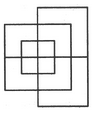Solution:

The figure may be labelled as shown.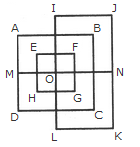The horizontal lines are IJ, AB, EF, MN, HG, DC and LK i.e. 7 in number.

The vertical lines are AD, EH, IL, FG, BC and JK i.e. 6 in number.

Thus, there are 7 + 6 = 13 straight lines in the figure.

QUESTION: 2

### Find the number of triangles in the given figure.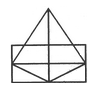Solution:

The figure may be labelled as shown.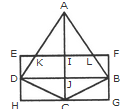The simplest triangles are AKI, AIL, EKD, LFB, DJC, BJC, DHC and BCG i.e. 8 in number.

The triangles composed of two components each are AKL, ADJ, AJB and DBC i.e. 4 in number.

The triangles composed of the three components each are ADC and ABC i.e. 2 in number.

There is only one triangle i.e. ADB composed of four components.

Thus, there are 8+ 4 + 2 + 1= 15 triangles in the figure.

QUESTION: 3

### Find the number of triangles in the given figure.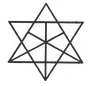Solution:

The figure may be labelled as shown.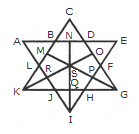The simplest triangles are ABL, BCD, DEF, FGP, PGH, QHI, JQI, KRJ and LRK i.e. 9 in number.

The triangles composed of two components each are OSG, SGQ, SPI, SRI, KSQ, KMS, FGH, JHI and JKL i.e. 9 in number.

There is only one triangle i.e. KSG which is composed of four components.

The triangles composed of five components each are NEI, ANI, MCG and KCO i.e. 4 in number.

The triangles composed of six components each are GMK and KOG i.e. 2 in number.

There is only one triangle i.e. AEI composed of ten components.

There is only one triangle i.e. KCG composed of eleven components.

Therefore, Total number of triangles in the given figure = 9 + 9+1 + 4 + 2+1 + 1 = 27.

QUESTION: 4

Find the number of triangles in the given figure.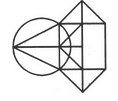Solution:

The figure may be labelled as shown.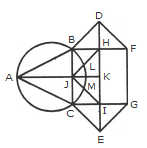The simplest triangles are ABJ, ACJ, BDH, DHF, CIE and GIE i.e. 6 in number.

The triangles composed of two components each are ABC, BDF, CEG, BHJ, JHK, JKI and CJI i.e. 7 in number.

There is only one triangle JHI which is composed of four components.

Thus, there are 6 + 7 + 1 = 14 triangles in the given figure.

QUESTION: 5

Find the number of triangles in the given figure.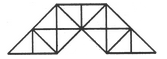Solution:

The figure may be labelled as shown.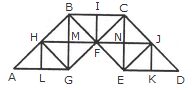The simplest triangles are AHL, LHG, GHM, HMB, GMF, BMF, BIF, CIF, FNC, CNJ, FNE, NEJ, EKJ and JKD i.e. 14 in number.

The triangles composed of two components each are AGH, BHG, HBF, BFG, HFG, BCF, CJF, CJE, JEF, CFE and JED i.e. 11 in number.

The triangles composed of four components each are ABG, CBG, BCE and CED i.e. 4 in number.

Total number of triangles in the given figure = 14 + 11 + 4 = 29.

QUESTION: 6

Count the number of triangles and squares in the given figure.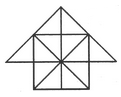Solution:

The figure may be labelled as shown.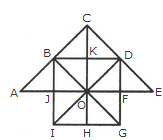Triangles:

The simplest triangles are JBO, BKO, KDO, DFO, FGO, GHO, HIO, IJO, ABJ, BCK, CKD and DEF i.e.12 in number.

The triangles composed of two components each are IBO, BDO, DGO, GIO, ABO, CDO, CBO, CBD and DEO i.e. 9 in number.

The triangles composed of four components each are IBD, BDG, DGI, GIB, ACO and COE i.e. 6 in number.

There is only one. triangle i.e. ACE composed of eight components.

Thus, there are 12 + 9 + 6 + 1 = 28 triangles in the given figure.

Squares:

The squares composed of two components each are BKOJ, KDFO, OFGH and JOHI i.e. 4 in number.

There is only one square i.e. CDOB composed of four components.

There is only one square i.e. BDGI composed of eight components.

Thus, there are 4 + 1 + 1 = 6 squares in the given figure.

QUESTION: 7

What is the minimum number of different colours required to paint the given figure such that no two adjacent regions have the same colour?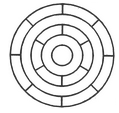Solution:

The figure may be labelled as shown.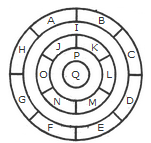The regions A, C, E and G can have the same colour say colour 1.

The regions B, D, F and H can have the same colour (but different from colour 1) say colour 2.

The region 1 lies adjacent to each one of the regions A, B, C, D, E, F, G and H and therefore it should have a different colour say colour 3.

The regions J, L and N can have the same colour (different from colour 3) say colour 1.

The regions K, M and O can have the same colour (different fromthe colours 1 and 3). Thus, these regions will have colour 2.

The region P cannot have any of the colours 1 and 2 as it lies adjacent to each one of the regions J, K, L, M, N and O and so it will have colour 3.

The region Q can have any of the colours 1 or 2.

Minimum number of colours required is 3.

QUESTION: 8

In the adjoining figure, if the centres of all the circles are joined by horizontal and vertical lines, then find the number of squares that can be formed.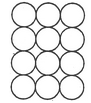Solution:

The figure may be labelled as shown.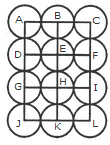We shall join the centres of all the circles by horizontal and vertical lines and then label the resulting figure as shown.

The simplest squares are ABED, BCFE, DEHG, EFIH, GHKJ and HILK i.e. 6 in number.

The squares composed of four simple squares are ACIG and DFLJ i.e. 2 in number.

Thus, 6 + 2 = 8 squares will be formed.

QUESTION: 9

Count the number of triangles and squares in the given figure.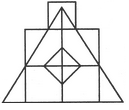Solution:

The figure may be labelled as shown.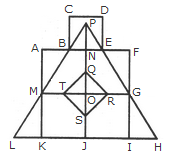Triangles :

The simplest triangles are BPN, PNE, ABM, EFG, MLK, GHI, QRO, RSO, STO and QTO i.e. 10 in number.

The triangles composed of two components each are BPE, TQR, QRS, RST and STQ i.e. 5 in number.

The triangles composed of three components each are MPO and GPO i.e. 2 in number.

The triangles composed of six components each are LPJ, HPJ and MPG i.e. 3 in number.

There is only one triangle LPH composed of twelve components.

Total number of triangles in the figure = 10 + 5 - 2 + 3 + 1 = 21.

Squares :

The squares composed of two components each are KJOM and JIGQ i.e. 2 in number.

The squares composed of three components each are ANOM, NFGO and CDEB i.e.3 in number.

There is only one square i.e. QRST composed of four components.

There is only one square i.e. AFIK composed of ten components.

Total number of squares in the figure = 2 + 3 + 1 + 1 = 7.

QUESTION: 10

Count the number of squares in the given figure.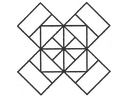Solution:

The figure may be labelled as shown.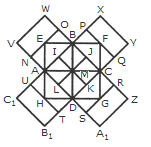The squares composed of two components each are BJMI, CKMJ, DLMK and AIML i.e. 4 in number.

The squares composed of three components each are EBMA, BFCM, MCGD and AMDH i.e. 4 in number.

The squares composed of four components each are VWBA, XYCB, ZA1DC and B1C1AD i.e. 4 in number.

The squares composed of seven components each are NOJL, PQKI, RSLJ and TUIK i.e. 4 in number.

There is only one square i.e. ABCD composed of eight components.

There is only one square i.e. EFGH composed of twelve components.

Total number of squares in the figure = 4 + 4 + 4 + 4 + 1 + 1 = 18.